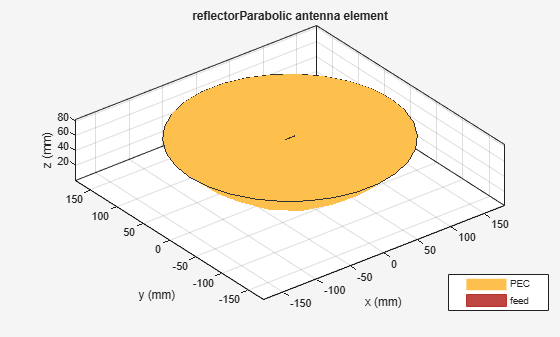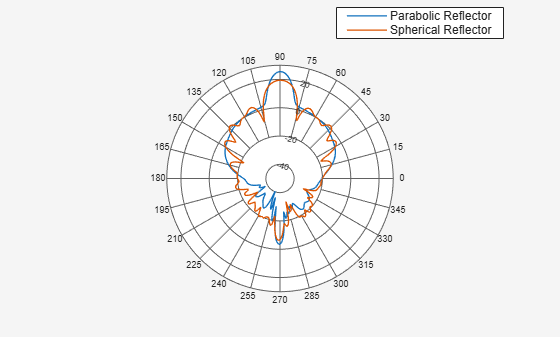Design and Analyze Curved Reflectors

This example shows how to design and analyze the curved reflectors. Curved reflectors are an upgraded version of rectangular reflectors. The curved surface on these reflectors reflects and focuses waves, which provides a stronger signal. Some of the notable characteristics of the curved reflectors are high gain, low cross-polarization, and reasonable bandwidth.

In this example, you will design and analyze two types of curved reflectors, the cylindrical and the spherical. Then, you will compare their radiation patterns with other types of of backing structures.

Design and Analyze Cylindrical Reflector

Cylindrical reflector uses a cylindrical shaped surface as a reflector. This reflector coexist with an antenna in various wireless transmission applications such as radar, general satellite communications, and in astronomical applications. The function of the reflector is to focus a beam of signal towards a specific direction for an enhanced antenna gain. The following properties of cylindrical reflector object, reflectorCylindrical are used in this example to define the cylindrical reflector and an exciter system.

• GroundPlaneLength Length of the ground plane in meters

• GroundPlaneWidth Width of the ground plane in meters

• Depth Perpendicular distance from origin to cylindrical reflector aperture in meters

GL = 0.3;
GW = 0.3;
D  = 0.075;
cref = reflectorCylindrical('GroundPlaneLength',GL,'GroundPlaneWidth',GW,'Depth',D);
cref.Tilt = 90;
cref.Exciter.TiltAxis = [1 0 0];
cref.Exciter.Tilt = 90;
show(cref)Plot the radiation pattern of the cylindrical reflector at 1 GHz.

pattern(cref,1e9)
view(-26,39)Design and Analyze Spherical Reflector

This type reflector are created by taking a cross section from the sphere and suitable for wide-angle scanning. The spherical reflector can provide beam scanning from a fixed reflecting surface without any distortion. This reduces scanning system cost in compared to a conventional reflector antenna systems. The cost reduction comes in eliminating the need to move the primary reflecting surface at all elevation angles. The following properties of the spherical reflector object, reflectorSpherical are used in this example to define spherical reflector and an exciter system.

• Depth Perpendicular distance between origin and the aperture of the antenna in meters

R = 0.15;
D = 0.15;
show(sref)Plot the radiation pattern of the spherical reflector backed dipole.

pattern(sref,10e9)Azimuth Plots of Rectangular and Cylindrical Reflectors

Plot the azimuth patterns of rectangular and cylindrical reflectors to compare the radiation characteristics of the two reflectors.

Create the rectangular reflector antenna object.

antR = reflector;
antR.Tilt = 90;
antR.Exciter.TiltAxis = [1 0 0];
antR.Exciter.Tilt = 90;
show(antR)Plot the azimulth pattern plots for the rectangular and the cylindrical reflectors and compare their results.

pa1 = patternAzimuth(antR,1e9,0,"Azimuth",0:-1:-360);
pa2 = patternAzimuth(cref,1e9,0,"Azimuth",0:-1:-360);
figure;
polarpattern(pa1);
hold on;
polarpattern(pa2);
hold off;
l = legend('Rectangular Reflector','Cylindrical Reflector');
l.Position = [0.6 0.8877 0.2996 0.0869];Cylindrical reflectors, on account of their curvature, provide higher gain compared to the rectangular reflectors.

Elevation Plots of Spherical and Parabolic Reflectors

Plot the elevation patterns of spherical and parabolic reflectors to compare the radiation characteristics of the two reflectors.

Create a parabolic reflector.

pref = reflectorParabolic;
show(pref)Compare the elevation pattern plots of the parabolic and the spherical reflectors.

pe1 = patternElevation(pref,10e9,0,'Elevation',0:360);
pe2 = patternElevation(sref,10e9,0,'Elevation',0:360);
figure
polarpattern(pe1)
hold on;
polarpattern(pe2)
l = legend('Parabolic Reflector','Spherical Reflector');
l.Position = [0.6 0.8877 0.2996 0.0869];The elevation pattern plot of the spherical reflector shows more peaks compared to the parabolic reflector. This means that a spherical reflector scans a wider area.

Reference

 Balanis, Constantine A. Antenna Theory: Analysis and Design. 3rd ed. Hoboken, NJ: John Wiley, 2005.

 Zali H.M., M.T.Ali, I.Pasya, N.A.Halili , H.Ja’afar, M.Hilmi. ''Design of a Cylindrical Parabolic Reflector on Monopole Plasma Antenna''. IEEE International RF and Microwave Conference (RFM), Penang, 2013, pp. 344-348.